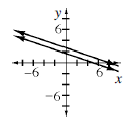### Home > CCA2 > Chapter 4 > Lesson 4.1.1 > Problem4-10

4-10.

Solve the system of equations shown at right.

$2x+6y=10\\x=8−3y$

1. Describe what happened when you tried to solve the system.

Use the Substitution Method.

1. Draw the graph of the system.1. How does the graph of the system explain what happened with the equations? Make your answer as clear and thorough as possible.

Where do the lines intersect?